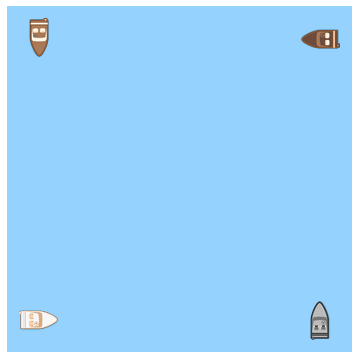# Four boats chasing

Four boats are on a large lake arranged so that they form the corners of a square of edge length $\ell = 1\text{ km}.$

At time zero, each boat starts moving with velocity $v_\textrm{boat} = \SI[per-mode=symbol]{10}{\kilo\meter\per\hour}$ such that its bow is always pointed directly toward its counterclockwise neighbor.

How long will the boats take to collide (in minutes)?×